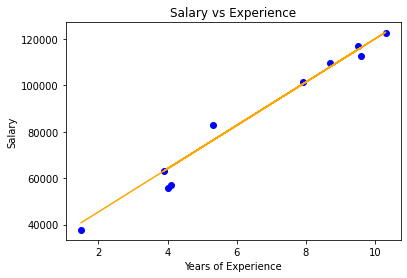Related Articles

# Implementation of Elastic Net Regression From Scratch

• Last Updated : 05 Sep, 2020

#### Prerequisites:

1. Linear Regression
3. Lasso & Ridge Regression

#### Introduction:

Elastic-Net Regression is a modification of Linear Regression which shares the same hypothetical function for prediction. The cost function of Linear Regression is represented by J.Here, m is the total number of training examples in the dataset.
h(x(i)) represents the hypothetical function for prediction.
y(i) represents the value of target variable for ith training example.


Linear Regression suffers from overfitting and can’t deal with collinear data. When there are many features in the dataset and even some of them are not relevant for the predictive model. This makes the model more complex with a too inaccurate prediction on the test set (or overfitting). Such a model with high variance does not generalize on the new data. So, to deal with these issues, we include both L-2 and L-1 norm regularization to get the benefits of both Ridge and Lasso at the same time. The resultant model has better predictive power than Lasso. It performs feature selection and also makes the hypothesis simpler. The modified cost function for Elastic-Net Regression is given below :Here, w(j) represents the weight for jth feature.
n is the number of features in the dataset.
lambda1 is the regularization strength for L-1 norm.
lambda2 is the regularization strength for L-2 norm.

#### Mathematical Intuition:

During gradient descent optimization of its cost function, added L-2 penalty term leads to reduces the weights of the model close to zero. Due to the penalization of weights, the hypothesis gets simpler, more generalized, and less prone to overfitting. Added L1 penalty shrunk weights close to zero or zero.  Those weights which are shrunken to zero eliminates the features present in the hypothetical function. Due to this, irrelevant features don’t participate in the predictive model. This penalization of weights makes the hypothesis more predictive which encourages the sparsity ( model with few parameters ).

Different cases for tuning values of lambda1 and lamda2.

1. If lambda1 and lambda2 are set to be 0, Elastic-Net Regression equals Linear Regression.
2. If lambda1 is set to be 0, Elastic-Net Regression equals Ridge Regression.
3. If lambda2 is set to be 0, Elastic-Net Regression equals Lasso Regression.
4. If lambda1 and lambda2 are set to be infinity, all weights are shrunk to zero

So, we should set lambda1 and lambda2 somewhere in between 0 and infinity.

#### Implementation:

It has 2 columns — “YearsExperience” and “Salary” for 30 employees in a company. So in this, we will train an Elastic-Net Regression model to learn the correlation between the number of years of experience of each employee and their respective salary. Once the model is trained, we will be able to predict the salary of an employee on the basis of his years of experience.

Code:

 # Importing libraries  import numpy as np  import pandas as pd  from sklearn.model_selection import train_test_split  import matplotlib.pyplot as plt  # Elastic Net Regression  class ElasticRegression() :          def __init__( self, learning_rate, iterations, l1_penality, l2_penality ) :                  self.learning_rate = learning_rate                  self.iterations = iterations                  self.l1_penality = l1_penality                  self.l2_penality = l2_penality              # Function for model training                  def fit( self, X, Y ) :                  # no_of_training_examples, no_of_features                  self.m, self.n = X.shape                  # weight initialization                  self.W = np.zeros( self.n )                  self.b = 0                  self.X = X                  self.Y = Y                  # gradient descent learning                          for i in range( self.iterations ) :                          self.update_weights()                      return self          # Helper function to update weights in gradient descent          def update_weights( self ) :                     Y_pred = self.predict( self.X )                  # calculate gradients                    dW = np.zeros( self.n )                  for j in range( self.n ) :                          if self.W[j] > 0 :                                  dW[j] = ( - ( 2 * ( self.X[:,j] ).dot( self.Y - Y_pred ) ) +                                                     self.l1_penality + 2 * self.l2_penality * self.W[j] ) / self.m                      else :                                  dW[j] = ( - ( 2 * ( self.X[:,j] ).dot( self.Y - Y_pred ) )                                                     - self.l1_penality + 2 * self.l2_penality * self.W[j] ) / self.m                 db = - 2 * np.sum( self.Y - Y_pred ) / self.m                   # update weights              self.W = self.W - self.learning_rate * dW              self.b = self.b - self.learning_rate * db                  return self          # Hypothetical function  h( x )           def predict( self, X ) :              return X.dot( self.W ) + self.b          # Driver Code  def main() :          # Importing dataset          df = pd.read_csv( "salary_data.csv" )      X = df.iloc[:,:-1].values      Y = df.iloc[:,1].values          # Splitting dataset into train and test set      X_train, X_test, Y_train, Y_test = train_test_split( X, Y,                                                                                                        test_size = 1/3, random_state = 0 )          # Model training          model = ElasticRegression( iterations = 1000,                                                       learning_rate = 0.01, l1_penality = 500, l2_penality = 1 )      model.fit( X_train, Y_train )          # Prediction on test set      Y_pred = model.predict( X_test )          print( "Predicted values ", np.round( Y_pred[:3], 2 ) )           print( "Real values      ", Y_test[:3] )          print( "Trained W        ", round( model.W, 2 ) )          print( "Trained b        ", round( model.b, 2 ) )          # Visualization on test set           plt.scatter( X_test, Y_test, color = 'blue' )          plt.plot( X_test, Y_pred, color = 'orange' )          plt.title( 'Salary vs Experience' )          plt.xlabel( 'Years of Experience' )          plt.ylabel( 'Salary' )          plt.show()          if __name__ == "__main__" :           main()

#### Output:

Predicted values  [ 40837.61 122887.43  65079.6 ]
Real values       [ 37731 122391  57081]
Trained W         9323.84
Trained b         26851.84Elastic-Net Model Visualization

Note: Elastic-Net Regression automates certain parts of model selection and leads to dimensionality reduction which makes it a computationally efficient model.

Attention reader! Don’t stop learning now. Get hold of all the important Machine Learning Concepts with the Machine Learning Foundation Course at a student-friendly price and become industry ready.

My Personal Notes arrow_drop_up